# A Guide to Linear Regression Using Scikit [With Examples]

Supervised learning algorithms are generally of two types: Regression and classification with the prediction of continuous and discrete outputs.

The following article will discuss linear regression and its implementation using one of the most popular machine learning libraries of python, the Scikit-learn library. Tools for machine learning and statistical models are available in the python library for classification, regression, clustering, and dimensionality reduction. Written in the python programming language, the library is built upon the NumPy, SciPy, and Matplotlib python libraries.

## Linear Regression

The linear regression performs the task of regression under the supervised learning method. Based on independent variables, a target value is predicted. The method is mostly used for forecasting and identifying a relationship between the variables.

In algebra, the term linearity means a linear relationship between variables. A straight line is deduced between the variables in a two-dimensional space.

If a line is a plot between the independent variables on the X-axis and the dependent variables on the Y-axis, a straight line is achieved through linear regression that best fits the data points.

The equation of a straight line is in the form of

Y = mx + b

Where, b= intercept

m= slope of the line

Therefore, through linear regression,

• The most optimal values for the intercept and the slope are determined in two dimensions.
• There is no change in the x and y variables as they are the data features and hence remain the same.
• Only the intercept and the slope values can be controlled.
• Multiple straight lines based on the values of slope and intercept might exist, however through the algorithm of linear regression multiple lines are fitted on the data points and the line with the least error is returned.

## Linear Regression with Python

For implementing linear regression in python, proper packages are to be applied along with its functions and classes. The package NumPy in Python is open source and allows several operations over the arrays, both single as well as multidimensional arrays.

Another widely used library in python is Scikit-learn which is used for machine learning problems.

Scikit-learN

The Scikit-learn library offers the developers algorithms based on both supervised and unsupervised learning. The open-source library of python is designed for machine learning tasks.

The data scientists can import the data, preprocess it, plot it, and predict data through the use of scikit-learn.

David Cournapeau first developed scikit-learn in 2007, and the library has seen growth since decades.

Tools provided by scikit-learn are:

• Regression: Includes the Logistic Regression and Linear regression
• Classification: Includes the method of K-Nearest Neighbors
• Selection of a model
• Clustering: Includes both K-Means++ and K-Means
• Preprocessing

• The learning and implementation of the library are easy.
• It is an open-source library and hence free.
• Machine learning aspects can be covered up including deep learning.
• It is a powerful and versatile package.
• The library has detailed documentation.
• One of the most used toolkits for machine learning.

### Importing scikit-learn

The scikit-learn has to be installed first through pip or through conda.

• Requirements: 64-bit version of python 3 with installed libraries NumPy and Scipy. Also for data plot visualization, matplotlib is required.

Installation command: pip install -U scikit-learn

Then verify whether the installation is complete

Installation of Numpy, Scipy, and matplotlib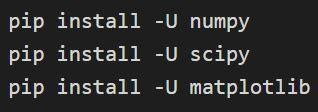Installation can be confirmed through: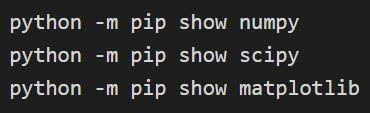### Linear regression through Scikit-learn

Implementation of the linear regression through the package scikit-learn involves the following steps.

• The packages and the classes required are to be imported.
• Data is required to work with and also to carry on the appropriate transformations.
• A regression model is to be created and fitted with the existing data.
• The model fitting data is to be checked to analyze if the model created is satisfactory.
• Predictions are to be made through the application of the model.

The package NumPy and the class LinearRegression are to be imported from the sklearn.linear_model.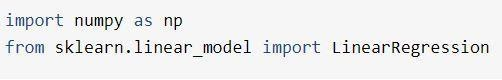Source

The functionalities required for sklearn linear regression are all present to finally implement linear regression. The sklearn.linear_model.LinearRegression class is used for performing regression analysis( both linear and polynomial ) and carrying out predictions.

For any machine learning algorithms and scikit learn linear regression, the dataset has to be imported first. Three options are available in Scikit-learn to get the data:

• Datasets like iris classification or the set of regression for housing price of Boston.
• Datasets of the real world can be downloaded from the internet directly through Scikit-learn predefined functions.
• A dataset can be generated randomly for matching against a specific pattern through the Scikit-learn data generator.

Whatever option is selected, the module datasets have to be imported.

import sklearn.datasets as datasets

### 1. The classification set of iris

The dataset iris is stored as a 2D array data field of n_samples * n_features. Its importation is carried out as an object of a dictionary. It contains all the necessary data along with the metadata.

The functions DESCR, shape and _names can be used to get descriptions and formatting of the data. Printing of function results will display the information of the dataset that could be needed while working on the iris dataset.

The following code will load the information of the iris dataset.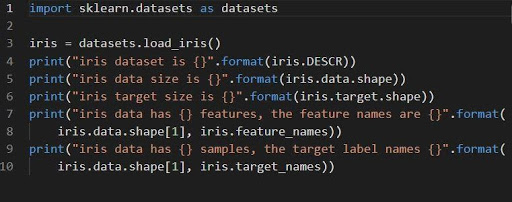Source

2. Generation of regression data

If there is no requirement for built-in data, then the data can be generated through a distribution that can be chosen.

Generating data of regression with a set of 1 informative feature and 1 feature.

X , Y = datasets.make_regression(n_features=1, n_informative=1)

The data generated is saved in a 2D dataset with the objects x, and y. The characteristics of the generated data can be changed through changing parameters of the function make_regression.

In this example, the parameters of the informative features and features are changed from a default value of 10 to 1.

Other parameters considered are the samples and targets where the number of target and sample variables tracked are controlled.

• The features that provide useful information to the algorithms of ML are referred to as the informative features while those that are unhelpful are referred to as on-informative features.

3. Plotting data

The data is plotted using the matplotlib library. First, the matplotlib has to be imported.

Import matplotlib.pyplot as plt

The above graph is plotted through the matplotlib through the code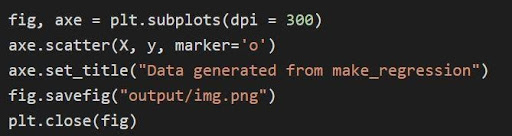Source

In the above code:

• The tuple variables are unpacked and saved as separate variables in line 1 of the code. Therefore, the separate attributes can be manipulated and saved.
• The dataset x, y is used to generate a scatter plot through line 2. With the availability of the marker parameter in matplotlib, the visuals are enhanced by marking the data points with a dot (o).
• The title of the generated plot is set through line 3.
• The figure can be saved as a .png image file and then the current figure is closed.

The regression plot generated through the above code is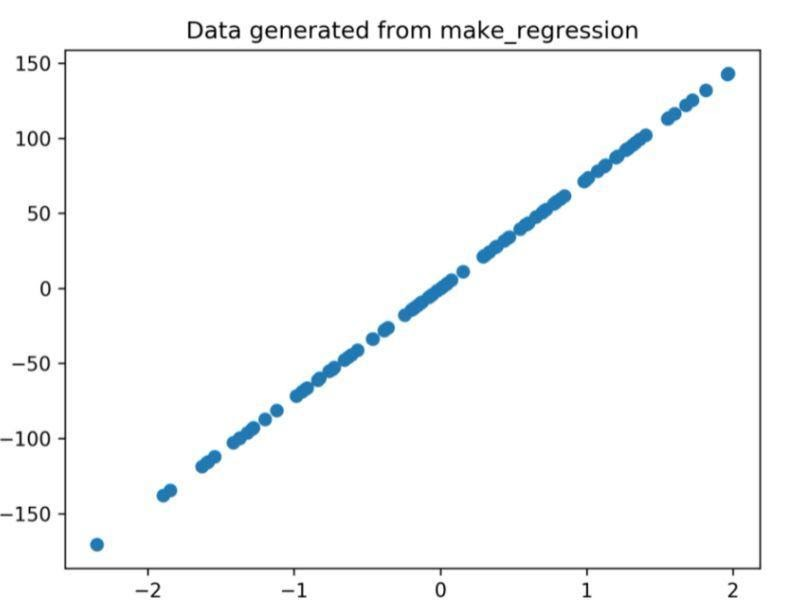Figure 1: The regression plot generated from the code above.

### 4. Implementing algorithm of linear regression

Using the sample data of the price of Boston housing, the algorithm of Scikit-learn linear regression is implemented in the following example. Like other ML algorithms, the dataset is imported and then trained using the previous data.

Linear method of regression is used by businesses, as it is a predictive model predicting the relationship between a numerical quantity and its variables to the output value with meaning having a value in reality.

When a log of earlier data is present, the model can be best applied as it can predict the future outcomes of what will be happening in the future if there is a continuation of the pattern.

Mathematically, the data can be fitted for minimizing the sum of all residuals that is existing between the data points and the value predicted.

The following snippet shows the implementation of sklearn linear regression.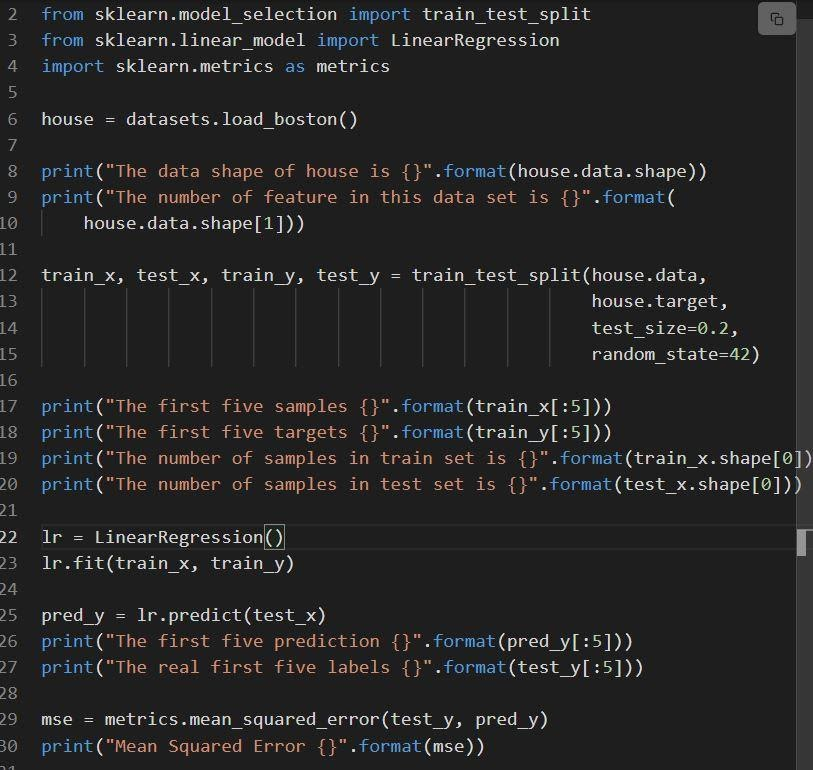The code is explained as:

• Dataset is split in line 12, i.e. the training set with 80% data and the set of the test with 20% data.
• Creation of a model of linear regression at line 23 and then trained at.
• The performance of the model is evaluated at linen 29 through calling mean_squared_error.

The sklearn linear regression plot is shown below:

Linear regression model of the Boston housing prices sample data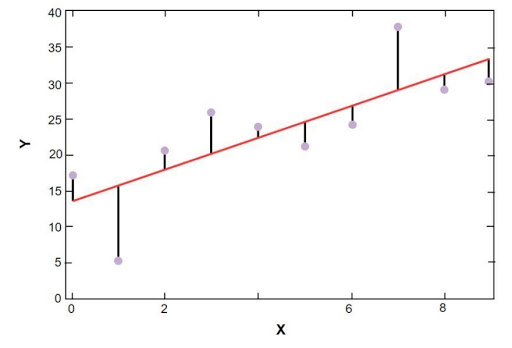Source

In the above figure, the red line represents the linear model that has been solved for the sample data of Boston housing price. Blue points represent the original data and the distance between the red line and the blue points represent the sum of the residual.  The goal of the scikit-learn linear regression model is to reduce the sum of the residuals.

## Conclusion

The article discussed linear regression and its implementation through the use of an open-source python package called scikit-learn. By now, you are able to get the concept of how to implement linear regression through this package. It is worth learning how to use the library for your data analysis.

If you have an interest in exploring the topic further, like the implementation of python packages in machine learning and AI-related problems, you can check the course Master of Science in Machine Learning & AI offered by upGrad. Targeting the entry-level professionals of 21 to 45 years, the course aims to train the students in machine learning through 650+ hour’s online training, 25+ case studies, and assignments. Certified from LJMU, the course offers the perfect guidance and job placement assistance. If you have any questions or queries, leave us a message, we will be happy to contact you.

## Lead the AI Driven Technological Revolution

EXECUTIVE PG PROGRAM IN MACHINE LEARNING AND ARTIFICIAL INTELLIGENCE
Apply Now

## 0 replies on “A Guide to Linear Regression Using Scikit [With Examples]”